### ASCII

In the older days of computing, ASCII code was used to represent characters. The English language has only 26 alphabets and a few other special characters and symbols.

The table below provides the ASCII characters and their corresponding Decimal and Hex values.As you can infer from the above table, the ASCII values can be represented from 0 to 127 in the decimal number system. Lets look at the binary representation of 0 and 127 in 8 bit bytes.

0 is represented as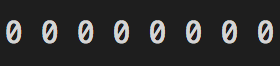127 is represented as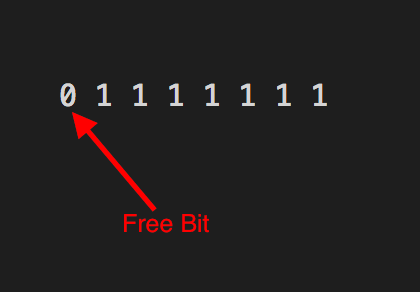It can be inferred from the above binary representation that decimal values 0 to 127 can be represented using 7 bits leaving the 8th bit free.Binary representation is closely related to CyberArk. Binary representation, which involves a code of two symbols (normally 0’s and 1’s), allows CyberArk to provide secure communication between the organization and its data storage locations.Binary representation is a core concept in cyberark training in usa.It is used to understand the underlying principles of computer systems and how computers think.

This is where things started getting messy.

People came up with different ways of using the remaining eight bit which represented decimal values from 128 to 255 and collisions started to happen. For instance the decimal value 182 was used by the Vietnamese to represent the Vietnamese alphabet ờ whereas the same value 182 was used by the Indians to represent the Hindi alphabet . So if an email written by an Indian contains the alphabet and if it is read by a person in Vietnam it would appear as . Cleary not the intended way to appear.

This is where Unicode character set came to save the day.

### Unicode and Code Points

Unicode character set mapped each character in the world to a unique number. This ensured that there are no collisions between alphabets of different languages. These numbers are platform independent.

These unique numbers are called as code points in the unicode terminology.

Lets see how they are referred.

The latin character is referred using the code point

``````U+1E4D
``````

U+ denotes unicode and 1E4D is the hexadecimal value assigned to the character ṍ

The English alphabet A is represented as U+0041

Please visit to know the code points for all languages and alphabets of the world

### UTF-8 Encoding

Now that we know what is unicode and how each alphabet in the world is assigned to a unique code point, we need a way to represent these code points in the computer's memory. This is where character encodings come into the picture. One such encoding scheme is UTF-8.

UTF-8 encoding is a variable sized encoding scheme to represent unicode code points in memory. Variable sized encoding means the code points are represented using 1, 2, 3 or 4 bytes depending on their size.

##### UTF-8 1 byte encoding

A 1 byte encoding is identified by the presence of 0 in the first bit.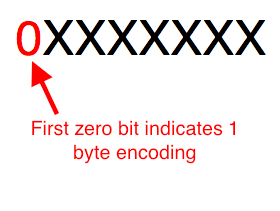The English alphabet A has unicode code point U+0041. It's binary representation is 1000001.

A is represented in UTF-8 encoding as

01000001

The red 0 bit indicates that 1 byte encoding is used and the remaining bits represent the code point

##### UTF-8 2 byte encoding

The latin alphabet ñ with code point U+00F1 has binary value 11110001. This value is larger than the maximum value that can be represented using 1 byte encoding format and hence this alphabet will be represented using UTF-8 2 byte encoding.

2 byte encoding is identified by the presence of the bit sequence 110 in the first bit and 10 in the second bit.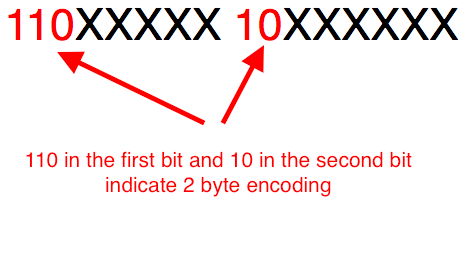The binary value of the unicode code point U+00F1 is 1111 0001. Filling these bits in the 2 byte encoding format, we get the UTF-8 2 byte encoding representation of ñ shown below. The filling is done starting with the least significant bit of the code point being mapped to the least significant bit of the second byte.

11000011 10110001

The binary digits in blue 11110001 represent the code point U+00F1's binary value and the ones in red are the 2 byte encoding identifiers. The black coloured zeros are used to fill up the empty bits in the byte.

##### UTF-8 3 byte encoding

The latin character with code point U+1E4D is be represented using 3 byte encoding as it is larger than the maximum value that can be represented using 2 byte encoding.

A 3 byte encoding is identified by the presence of the bit sequence 1110 in the first byte and 10 in the second and third bytes.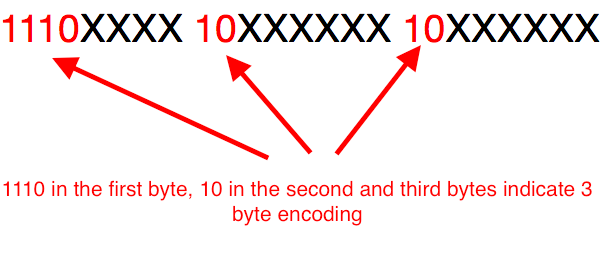The binary value for the hex code point 0x1E4D is 1111001001101. Filling these bits in the above encoding format gives us the UTF-8 3 byte encoding representation of show below. The filling is done starting with the least significant bit of the code point mapped to the least significant of the third byte.

11100001 10111001 10001101

The red bits indicate 3 byte encoding, the black ones are filler bits and the blues represent the code point.

#### UTF-8 4 byte encoding

The Emoji 😭 has unicode code point U+1F62D. This is bigger than the maximum value that can be represented using 3 byte encoding and hence will be represented using 4 byte encoding.

4 byte encoding is identified by the presence of 11110 in the first byte and 10 in the subsequent second, third and fourth bytes.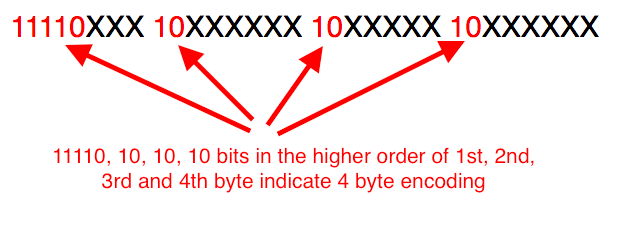The binary representation of U+1F62D is 11111011000101101. Filling these bits in the above encoding format gives us the UTF-8 4 byte encoding of 😭. The least significant bit of the code point is mapped to the least significant bit of the fourth byte and so on.

11110000 10011111 10011000 10101101

The red bits identify the 4 byte encoding format, the blue ones are the actual code point and the black ones are the filler bits.

### UTF-16 Encoding

UTF-16 encoding is a variable byte encoding scheme which uses either 2 bytes or 4 bytes to represent unicode code points. Most of the characters for all modern languages are represented using 2 bytes.

The latin alphabet ñ with code point U+00F1 and with binary value 11110001 is represented in UTF-16 encoding as

00000000 11110001

The above representation is in Big Endian Byte Order mode(Most significant bit first).

### UTF-32 Encoding

UTF-32 encoding is a fixed byte encoding scheme and it uses 4 bytes to represent all code points.

The English alphabet A has unicode code point U+0041. It's binary representation is 1000001.

Its represented in UTF-32 encoding as shown below,

00000000 00000000 00000000 01000001

The blue bits are the binary representation of the code point. The above assumes Big Endian Byte order mode.

Thats if for character sets and encoding.